SCHOLASTIC APTITUDE TEST 1997

MATHEMATICS

 Time: Two Hours Max. Marks: 60 (8.30 AM - 10.30 AM)

NOTE:-

1. Answers must be written in English or the medium of instruction of the candidate in High school.
2. Answer to each question should begin on a fresh page, and rough work must be enclosed with answer book.
3. Attempt all questions.
4. There is no negative marking.
5. Answer all parts of a question at one place.
6. Use of calculators, slide rule, graph paper and logarithmic, trigonometric and statistical tables is not permitted

Note:- All answers to questions in Section-A, Section-B, Section-C must be supported by mathematical arguments unless explicitly exempted.

SECTION-A

I. This section has Five questions. Each question is provided with five alternative answers. Only one of them is the correct answer. Indicate the correct answer by A,B,C,D,E. Order of the questions must be maintained.                                                     (5 x 2 =10 MARKS)

1. n1, n2, ….., n1997 are integers, not necessarily distinct.

X =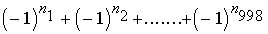;

Y =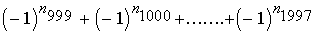A) (-1)X = 1, (-1)Y = 1 B) (-1)X = 1, (-1)Y = -1 C) (-1)X = -1, (-1)Y = 1 D) (-1)X = -1, (-1)Y = -1 E) none of these

2. I and S are the incentre and circumcentre of D ABC. Let Ð AIB = a and Ð ASB = b . Then

 A) a is acute and nothing can be said about b B) a is obtuse and nothing can be said about b C) nothing can be said about a and b is acute D) nothing can be said about a and b is obtuse E) none of these

3. Treating all similar polygons as indistinct, the number of convex decagons such that four of its angles are acute is

 A) 0 B) infinitely many C) 1 D) 10 E) none of these

4. The first hundred natural numbers are written down. Ni denotes the number of times the digit i appears. Then

 A) N0=12, N1=20, N2=20 B) N0=11, N1=20, N2=20 C) N0=11, N1=21, N2=20 D) N0=12, N1=20, N2=19 E) none of these

5 Consider the decimal 0.111010100010………, where the digit in the nth decimal place is 1 if either n=1 or n is a prime. Then the decimal

 A) terminates B) has only finite number of zeros C) recurs D) denotes a rational number E) denotes an irrational number

SECTION-B

II. This section has Five questions. In each question a blank is left. (5 x 2 =10 MARKS)

Fill in the blank.

1. k is a given real constant. f(x) is a real quadratic in x such that f(x+k) = f(-x). The coefficient of x2 is 1. Then f(x) is of the form ___________________

2. A is a 2x2 real matrix such that A2 = 0 and none of its elements is a zero. Then A is of the form __________________________

3. If X is a finite set, let P(X) denote the set of all subsets of X and let n(X) denote the number of elements in X. If for two finite sets A,B, n(P(A)) = n(P(B)) + 15, then n(B) = _____________,        n(A) = ___________.

4. p is a natural number such that no term in the expansion of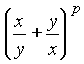is independent of x. The p is of the form ______________________.

5. The circle C1 has centre at (2,3) and radius 3. The circle C2 has centre at (-1,4) and radius 5. The number of common tangents they possess is ________________

SECTION-C

III. This section has Five questions. Each question has a short answer. Elegant solutions will be rewarded.                                                                                 (5 x 2 =10 MARKS)

1. Sketch the graph of the region 0 < x < y < 1 on plain paper and describe it in words.

(No verbal argument is required)

2. Find the value of x3+y3+z3-2xyz, if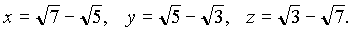3. The remainder when x5+kx2 is divided by (x-1) (x-2) (x-3) contains no term in x2. Find k, without performing division.

4.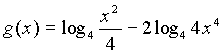if x ¹ 0. Determine the function f(x) and constants a,b

such that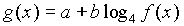5. How do you construct a tangent to a circle through a given external point? Justify your answer.

SECTION-D

IV. This section has six questions. These questions are long answer questions. Elegant solutions will be rewarded                                                            (6 x 5 =30 MARKS)

1. If x3+px+q = (x-a ) (x-b ) (x-g ), then rewrite the polynomial (b g x+b +g ) (g a x+g +a ) (a b x+a +b ), in terms free of a ,b ,g .

2. If x+y+z = 15, xy+yz+zx = 72, prove that 3 £ x £ 7.

3. If the sides and the area of a triangle have integral measures prove that its perimeter must have even integral measure.

4. m and n are positive integers such that (i) m < n, (ii) they are not relatively prime, (iii) their product is 13013. Find their g.c.d. and hence determine all such ordered pairs (m,n).

5. Two circles 6cm, 18cm in diameter are externally tangent. Compute the area bounded by the circles and an external common tangent.

6. From any point P within a triangle ABC, perpendiculars PA' , PB' , PC' are dropped on the sides BC, CA, AB; and circles are described about PA' B' , PB' C' , PC' A' . Show that the area of the triangle formed by joining the centres of these circles is one-fourth of the area of  DABC.